﻿ 蓝凌新一代数字OA_打造一站式数字化办公平台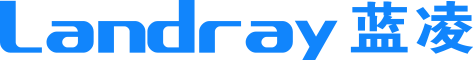### 10+门户、千人千面一站式数字化OA办公更高效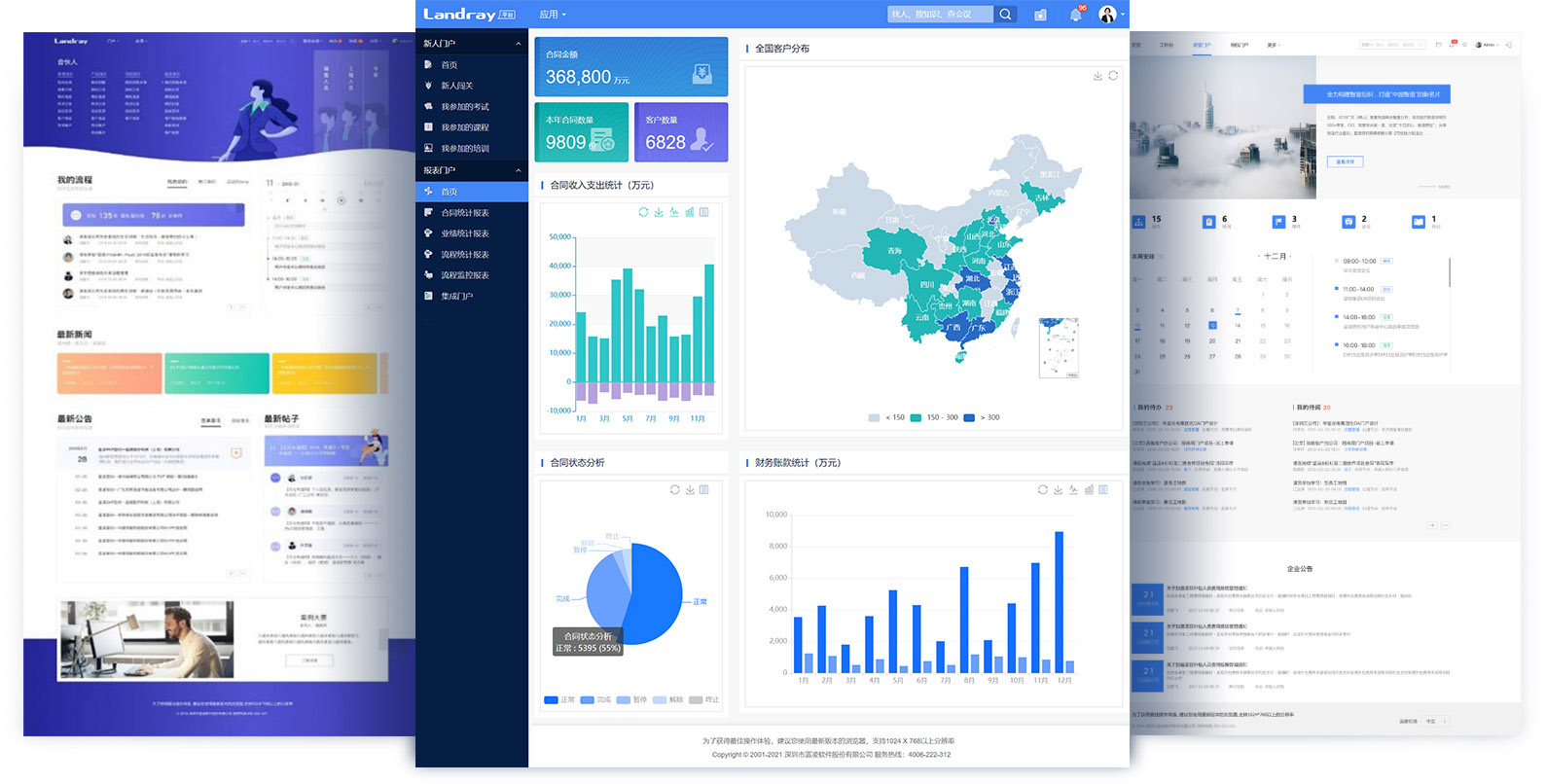•个人门户

•信息门户

•营销门户

•新人门户

•报表门户

•党建门户

•财务门户

•人事门户

•IT门户

•行政门户

### 12大机制、1000+模板让企业OA流程审批更灵活、高效

•招聘申请

•入职申请

•转正申请

•转岗申请

•离职申请

•绩效评审

•请假审批

•出差申请

•公告审批

•用印申请

•证照借用

•物品领用

•用车申请

•档案借阅

•访客审批

•会议申请

•采购申请

•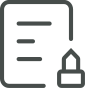合同审批

•供应商评审

•合同归档

•维修申请

•工作周报

•月度计划

•资产盘点

•数据报送

•物料申领

•清单确认

•备货计划

•广告发布

•投标申请

•权限申请

•费用报销

•消息

•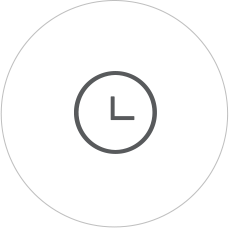时间

•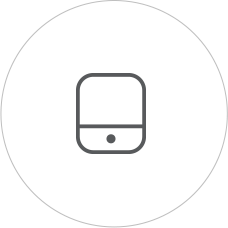移动

•发布

•订阅

•催办

•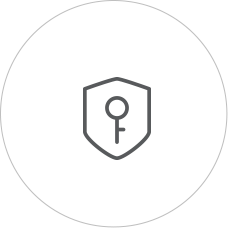权限

•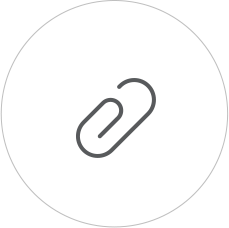附件

•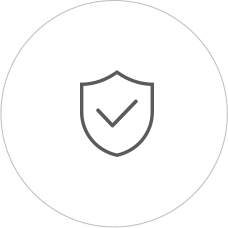授权

•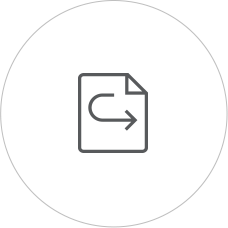传阅

•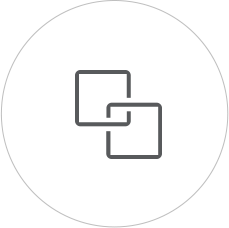关联

•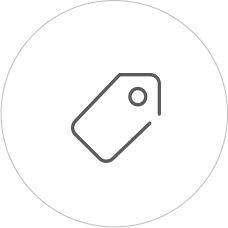标签

### 6大集成打造数字化闭环应用、数据互联支撑业务增长

•业务集成

•流程集成

•邮件集成

•报表集成

•组织集成

•登录集成

### 100+OA应用开箱即用让企业“人财物事”协同办公更高效

•流程管理

•新闻管理

•会议管理

•任务管理

•论坛管理

•工作沟通

•公文管理

•时间管理

•调查管理

•工作总结

•规范制度

•用品管理

•车辆管理

•固资管理

•合同管理

•客户台账

•员工活动

•知识地图

•投票管理

•图表中心

•流程分析

•招聘管理

•绩效管理

•人事档案

### 1小时搭建一个应用低代码让更多新应用触手可得

• UI、移动组件丰富，调用更方便
• 可视化表单、流程设计，门槛低
• 规则配置化，灵活应对业务变化
• 动态生成多图表，支撑数据分析
• 精细化权限设计，让应用更安全
• 支持全面移动化，应用随时随地